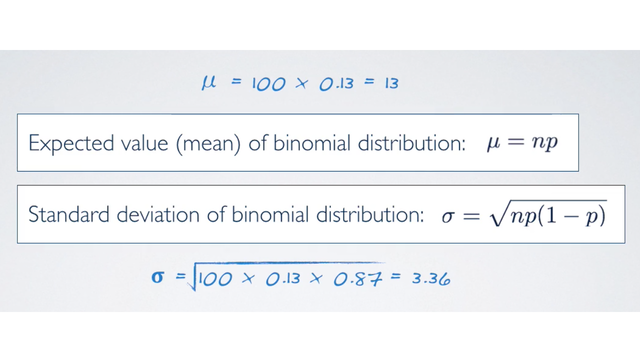### Question from an Example

Among a random sample of 100 employees, how many would you expect to be engaged at work?

Worldwide only 13% of employees are engaged at work, so p = 0.13

The expected number of engaged employees is:

`n × p = 100 × 0.13 = 13`

More formally, the Expected Value (mean) of binomial distribution is:

`Expected Value (mean) of binomial distribution = μ = np`

However, this doesn't mean that in every random sample of 100 employees exactly 13 will be engaged at work. In some examples, this value will be fewer, and in others, more. So how much would we expect this value to vary?

### Standard Deviation of Binomial Distribution

As usual, we can quantify the variability around the mean using the standard deviation. And for Binomial Distribution, the standard deviation is defined as the square root of np(1-p):So, plug in the values from the survey, we would expect:

`sqrt(100 × 0.13 × 0.87) = 3.36. `

This means that 13 out of 100 employees are expected to be at engaged at work, give or take approximately 3.36.

• N/A